Related Articles

# Sorting contents of a Data Frame in Julia

• Last Updated : 25 Mar, 2021

Sorting is a technique of storing data in sorted order. Sorting can be performed using sorting algorithms or sorting functions. to sort in a particular dataframe then extracting its values and applying the sorting function would easily sort the contents of a particular dataframe. Julia provides various methods that can be used to perform the sorting of DataFrames.

Remember to add the following package before starting i.e DataFrames with help of below code:

```using Pkg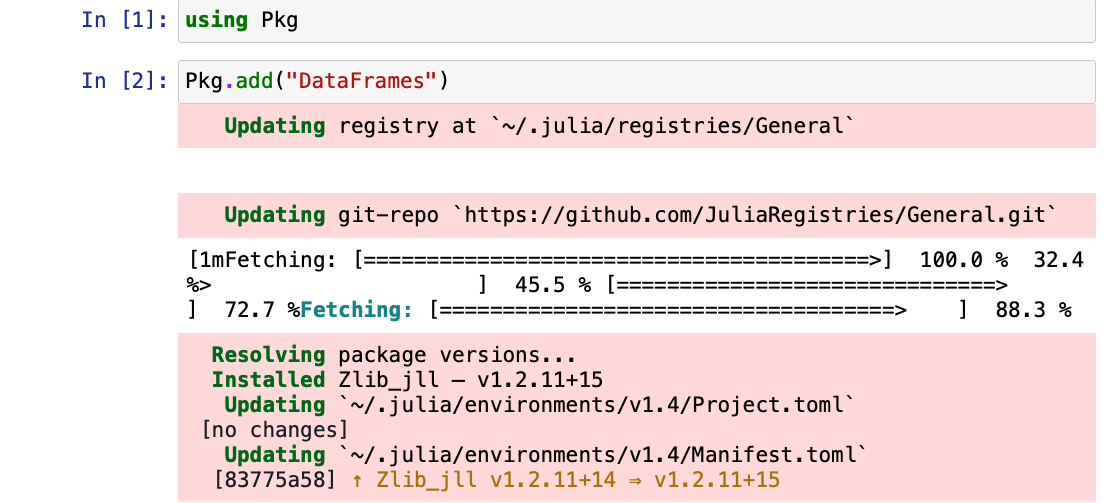#### Methods for Sorting

Julia provides some methods for sorting data of a DataFrame:

• sort() function: This function returns a sorted array or sorted copy of an array
• sort() function passing an algorithm: This function returns a sorted array with the help of an applied algorithm
• sortperm() function: This function returns a list of indices that one can use on the collection to produce a sorted collection.
• partialsortperm() function: This function partially sorts the algorithm up to a particular rang or permutation.
• sort() function with rev=True: This function will sort the content of the dataframe into descending order.
• sort!(): This function passing the dimension, this function can sort multidimensional arrays of DataFrames.
• partialsortperm(): This function returns a partial permutation DataFrame’s column of the vector

#### Method 1:  Use of sort() Function

sort() Function in Julia is the most basic sorting method that can be used to sort data of a dataframe.

Approach:

• First, you can create the dataframe
• The sort() function has arguments like the vector and the order in which the columns need to be sorted.

## Julia

 `# Creating a dataframe``df1 ``=` `DataFrame(b ``=` `[:Hi, :Med, :Hi, :Low, :Hi],``               ``x ``=` `[``"A"``, ``"E"``, ``"I"``, ``"O"``,``"A"``],``               ``y ``=` `[``6``, ``3``, ``7``, ``2``, ``1``],``               ``z ``=` `[``2``, ``1``, ``1``, ``2``, ``2``])` `# Method1``sort(df1,[:z,:y]) ``# sorted z then y`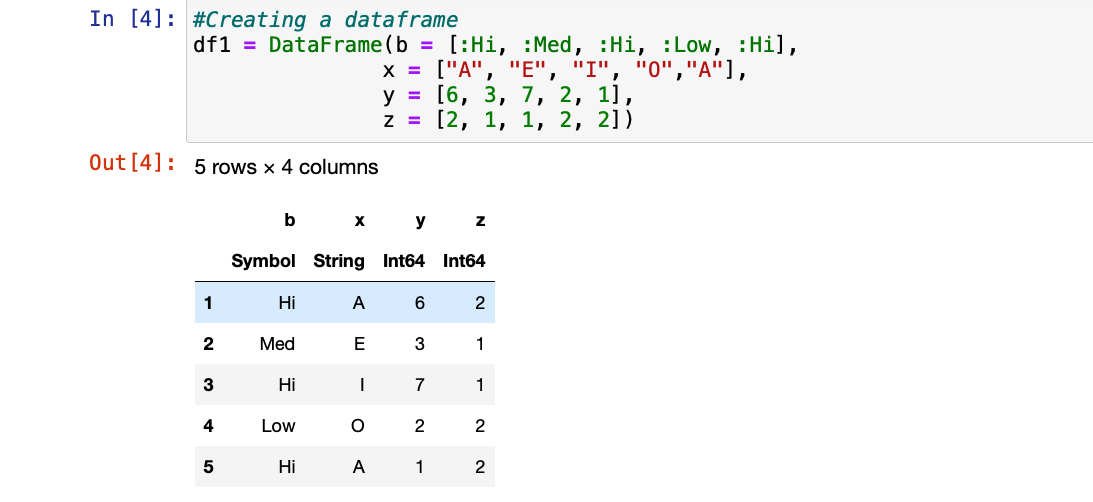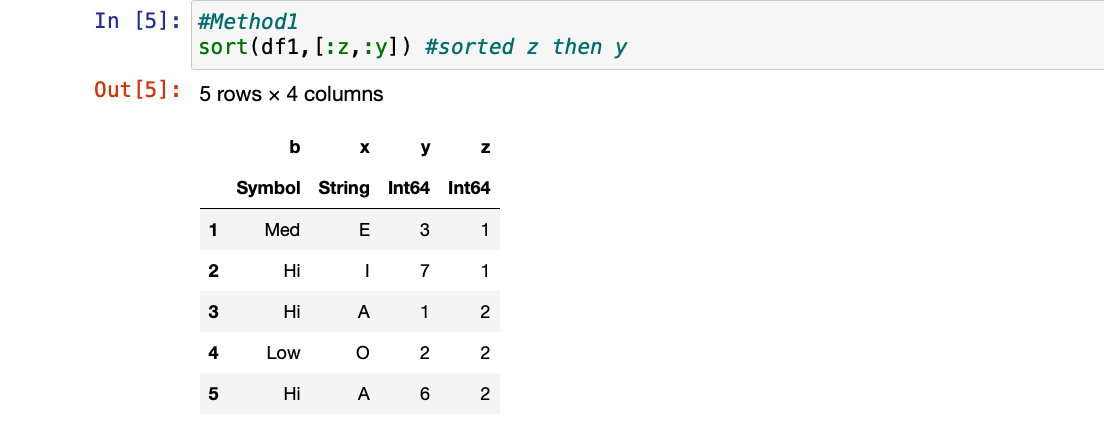#### Method 2: Sort using Quicksort algorithm

Julia allows passing the algorithm type to sort() function to sort the column. sort(dataframe.columnheader; alg=QuickSort) function takes column name and algorithm type as an argument.

Approach:

• Here, the sort() function is applied to a specific column.
• It is passed as an argument in the sort function
• Then the algorithm with which you want to sort the particular column is also passed as an argument
• Store the returned value of this function in a separate variable
• Then update in the particular column

## Julia

 `# Method2 Algorithm(Quicksort)``# Sorting a particular column and storing it in s``s ``=` `sort(df1.y; alg ``=` `QuickSort)` `# Now giving the value of s to the dataframe's y header``df1.y ``=` `s``df1 ``# printing the sorted y`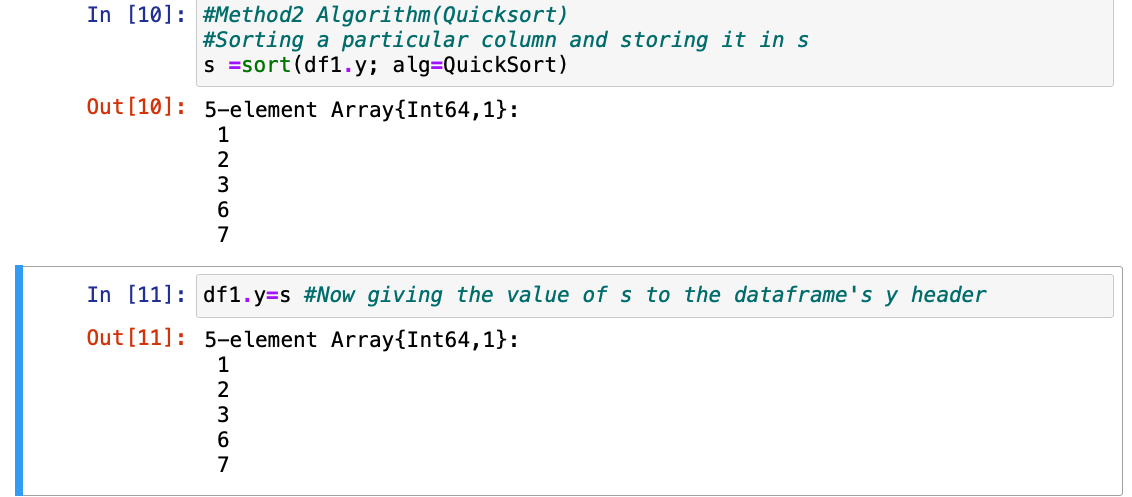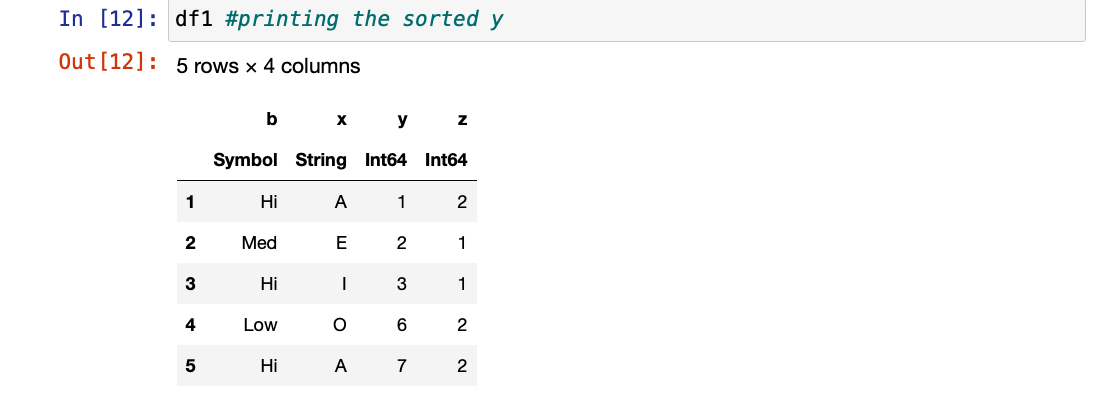#### Method 3: Sort using Partial QuickSort algorithm

sort(dataframe.columnheader; alg=PartialQuickSort(range)) function is passed with PartialQuickSort algorithm to sort the column upto a certain limit which is passed in the algorithm.

Approach:

• Here, the sort() function is applied to a specific column.
• It is passed as an argument in the sort function
• Then the algorithm(PartialQuickSort) with which you want  to sort the particular column is also passed as an argument
• Store the returned value of this function in a separate variable
• Then update in the particular column

## Julia

 `# Method3 Algorithm(PartialQuickSort)``# If we want sort a column upto a certain number``B ``=` `3``t ``=` `sort(df1.z; alg ``=` `PartialQuickSort(B))` `# passing the t variable in the dataframe``df1.z ``=` `t``df1`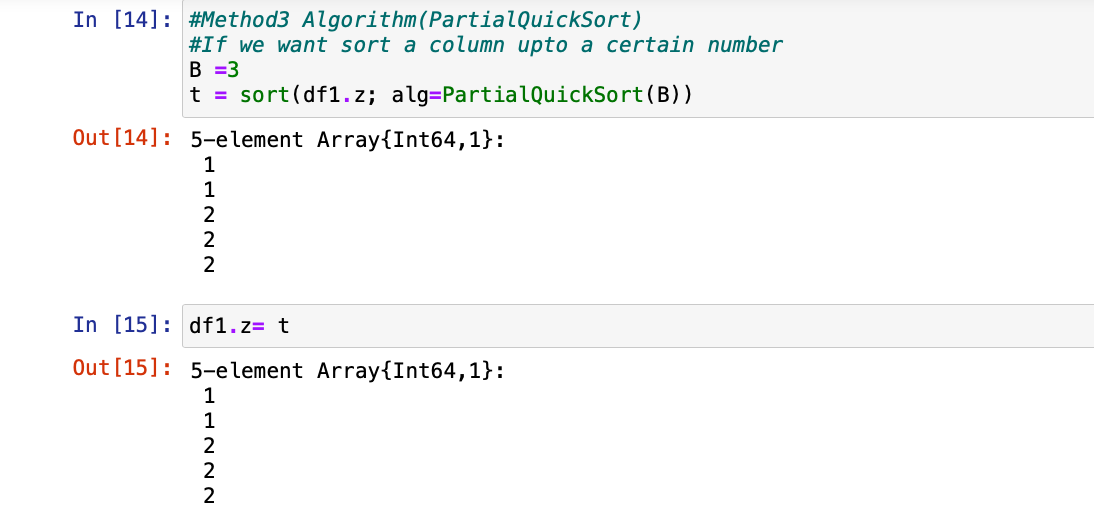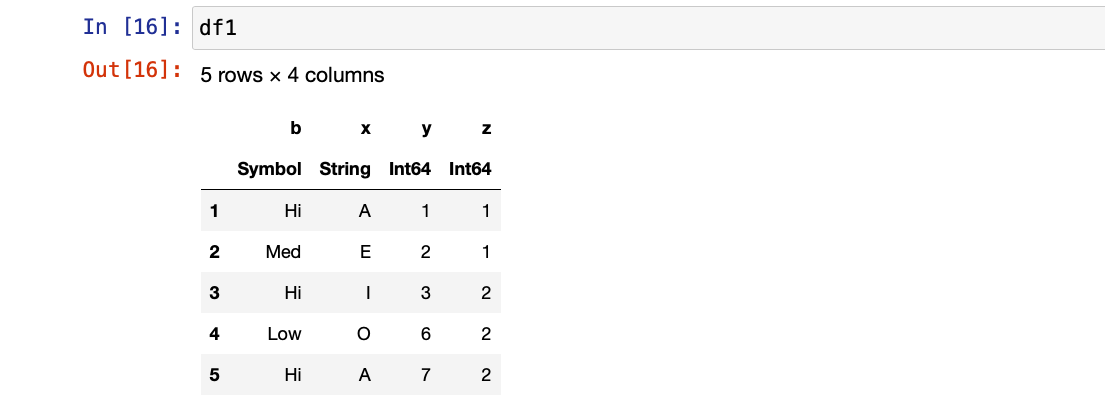#### Method 4: Use of sortperm() function

sortperm() function is passed with the column name, to sort the column and return indexes of the sorted column.

Approach:

• First store the particular column in which you want to apply this sorting in a separate variable
• Apply the sortperm() function and pass the variable as argument this will return the sorted indexes of the particular column and store the returned indexes in a separate variable
• Then traverse using the for loop in the variable where the indexes are stored
• Print using the for loop and pass the index in the variable where the particular column was stored.

## Julia

 `# Method4``r ``=` `df1.y` `# returned indexes of the elements``k ``=` `sortperm(r)` `# traversing through indices``for` `i ``in` `k``    ``println(r[i]) ``end`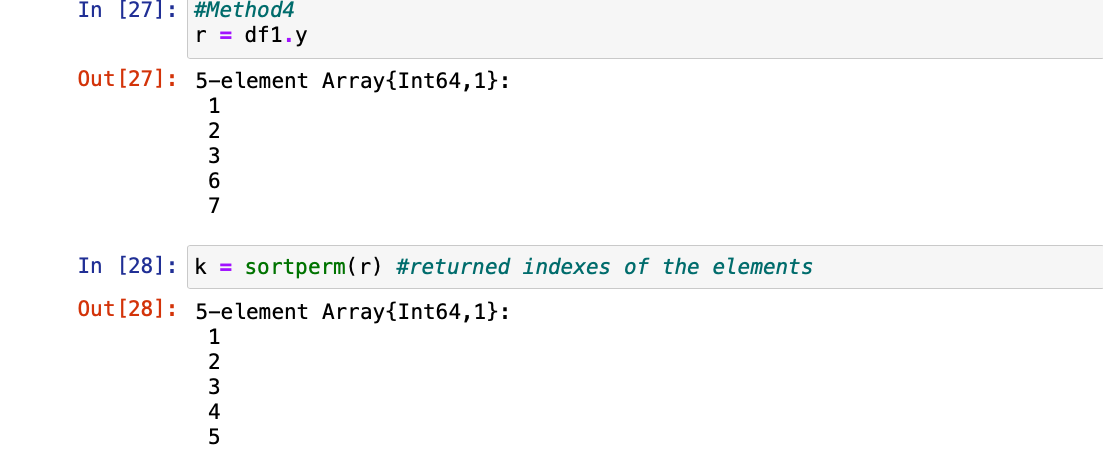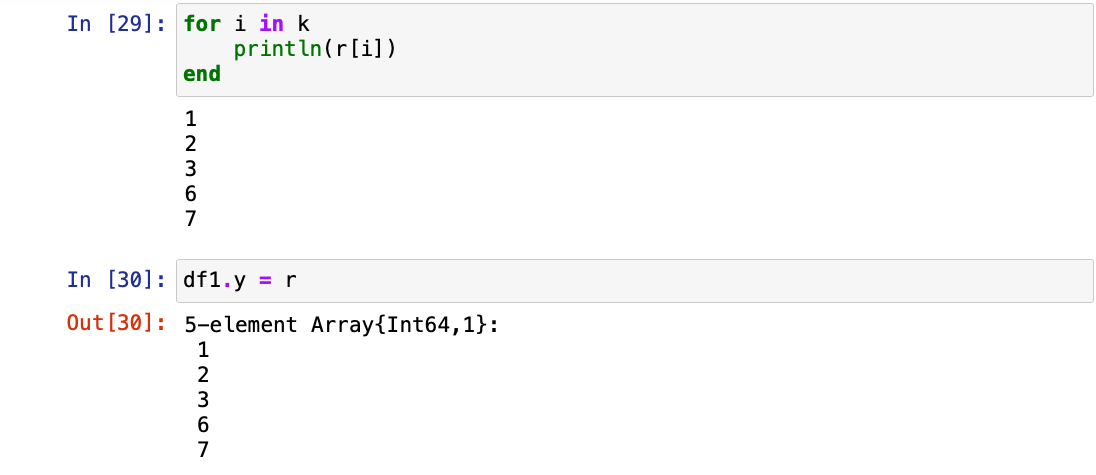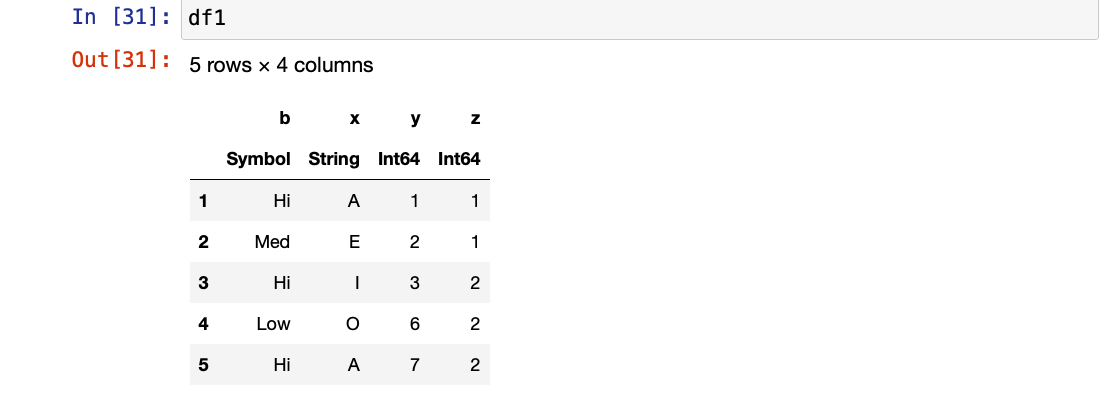#### Method 5: Sort using Insertion sort Algorithm

sort(dataframe.column;alg=InsertionSort) function is passed with InsertionSort algorithm to sort the column up to certain limit which is passed in the algorithm.

Approach:

• Creating a new dataframe  and applying the sort() function
• This time the algorithm used is insertion sort
• Then the algorithm(InsertionSort) with which you want  to sort the particular column is also passed as an argument
• Store the returned value of this function in a separate variable
• Then update in the particular column

## Julia

 `# Created new dataframe as df2``df2 ``=` `DataFrame(x ``=` `[``11``,``12``, ``13``, ``10``, ``23``],``                ``y ``=` `[``6``, ``3``, ``7``, ``2``, ``1``],``                ``z ``=` `[``2``, ``1``, ``1``, ``2``, ``2``])``# Method5``s2 ``=` `sort(df2.x; alg ``=` `InsertionSort)` `# now update the df2.x``df2.x ``=` `s2``df2`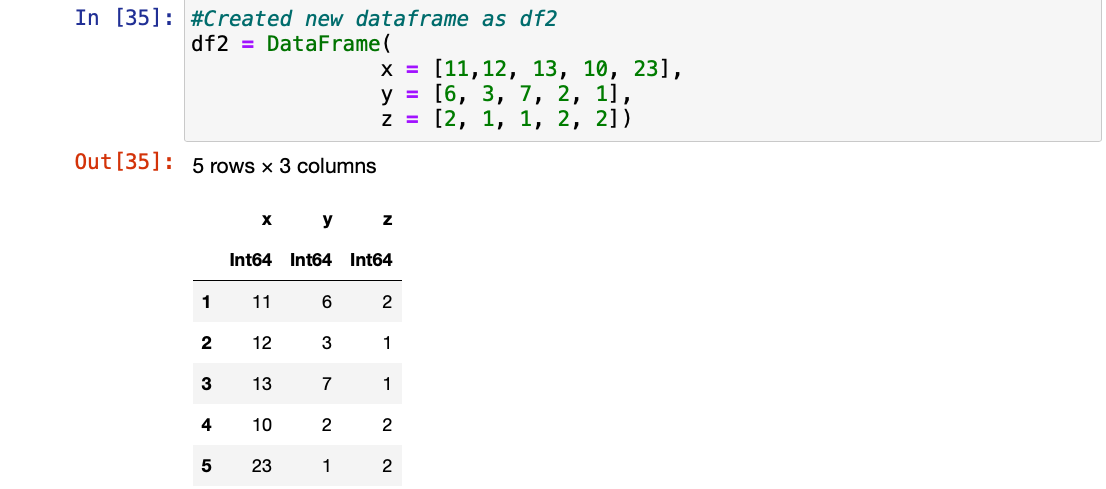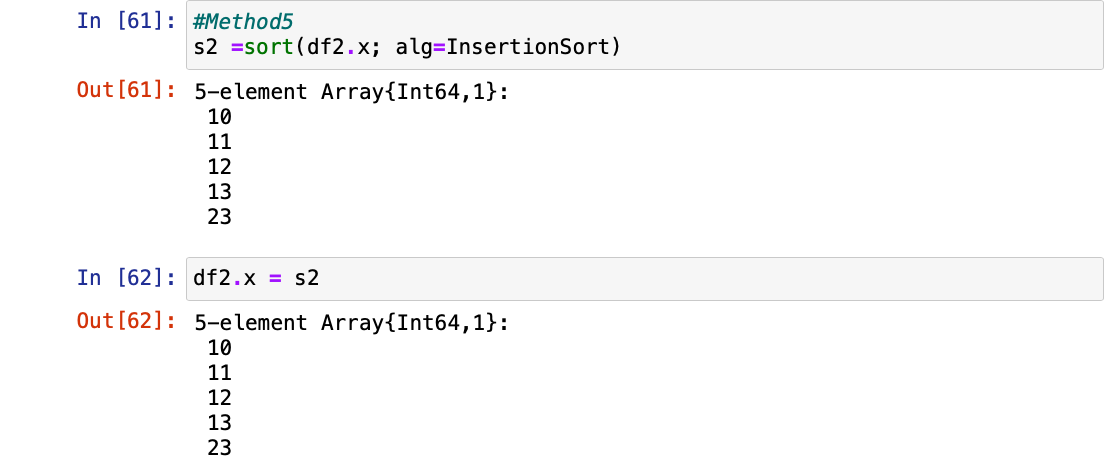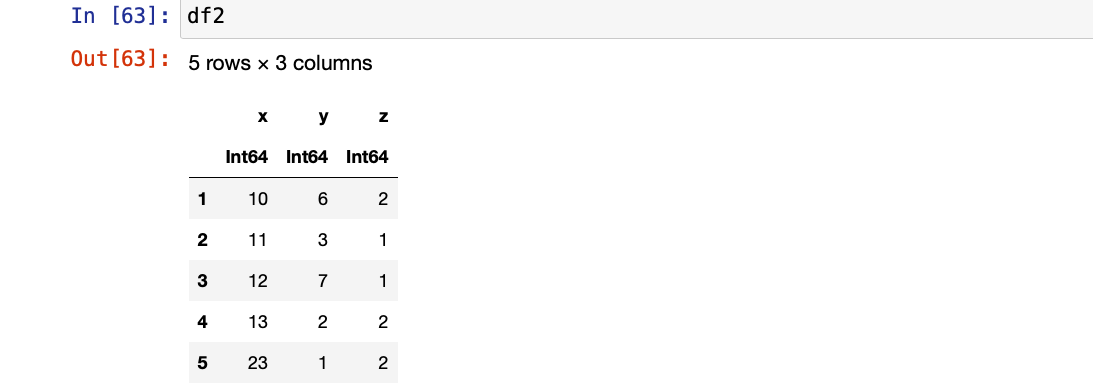Method 6: Use of partialsortperm() function

partialsortperm(dataframe.column, range) function is an advanced form of sortperm() function which will return the indexes of the values which are in range. This partially sorts the column.

Approach:

• Storing the particular column which needs to be sorted in another variable
• Applying the partialsortperm() function passing the vector and the range till which it needs to be sorted
• Finally, we can update with the help of passing the result into the particular DataFrame’s column
• Now printing the dataframe would simply print with updated value

## Julia

 `# Method6``a ``=` `df2.y``a ``=` `a[partialsortperm(a, ``1``:``5``)]``a`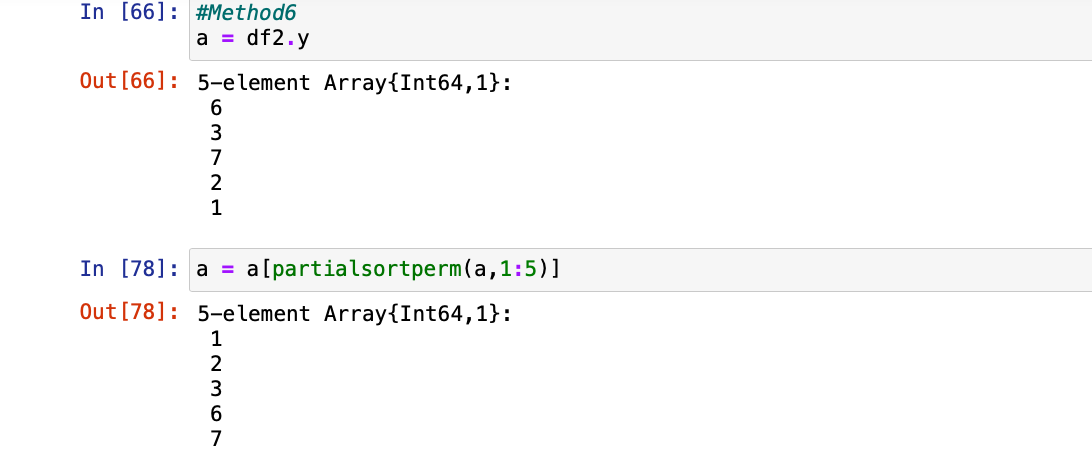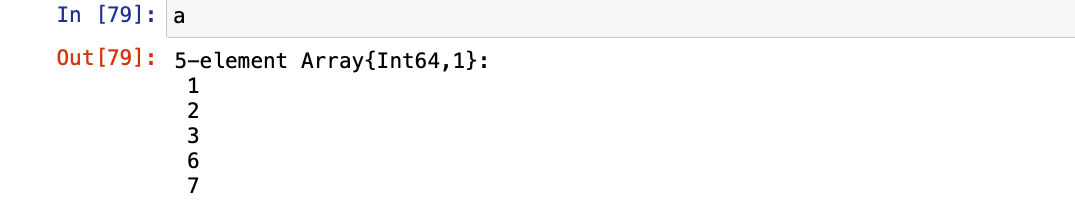#### Method 7: Sorting in Descending order

sort(dataframe,rev=True) function is passed with dataframe and rev variable to sort the column. This function basically reverses or gives a descending order of the column passed.

Approach:

• Sorting the dataframe’s particular column in the descending order using the sort() function
• First storing the particular column in the variable
• And applying the sort() function and passing the reverse of the particular column as rev = true
• This now will sort in the descending order
• At last, updating the dataframe by passing the variable into the dataframe

## Julia

 `# Method7``s2 ``=` `sort(df2, rev``=``true)``df2 ``=` `s2 ``#updating the whole dataframe``df2`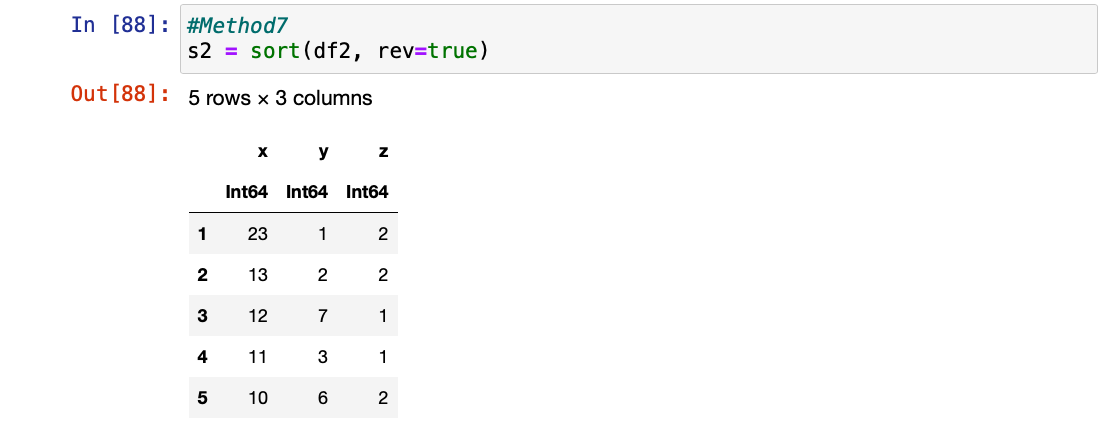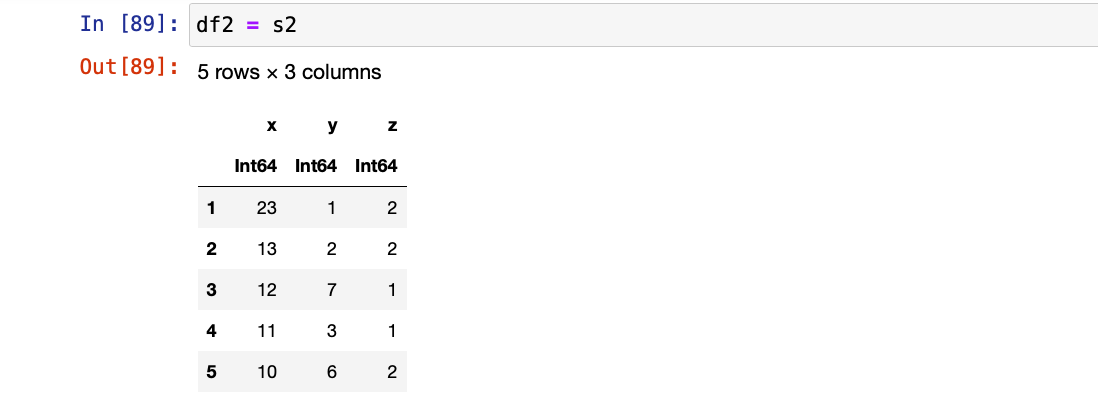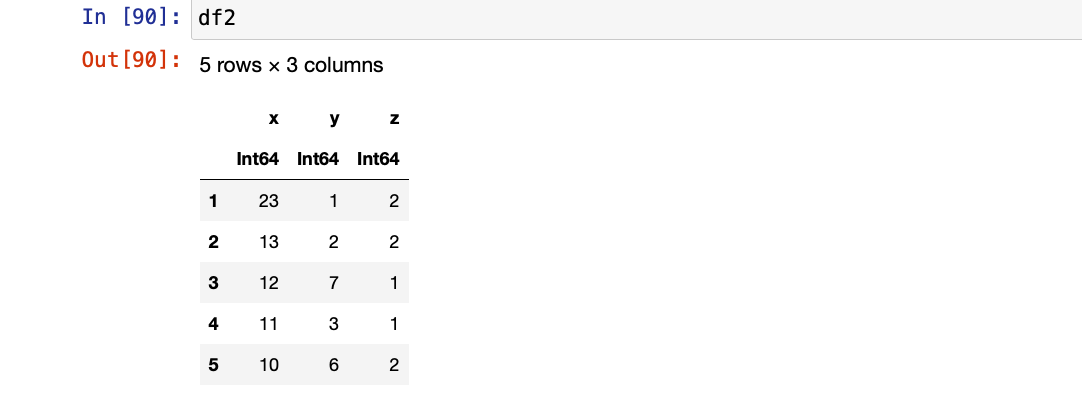#### Method 8: Use of sort()! function

sort!(vector,dim) function is passed with dataframe and dimension in which we want to sort the column (dimension means dim=1 means row and dim=2 means column).

Approach:

• The function for now applying the sort with user’s choice to either sort by row or column
• Sorting by row is done by passing  vector into the sort!() function
• Also, we need to pass the dim=1 which means to traverse row-wise
• This function will print the sorting in the row manner
• Now applying  the same function by just passing dim=2 to sort in column manner.
• This now would print the sorted vector in the column manner.

## Julia

 `# Method8``B ``=` `[``4` `3``; ``1` `2``]``sort!(B, dims ``=` `1``); B ``# sorting through row``sort!(B, dims ``=` `2``); B ``# sorting through column`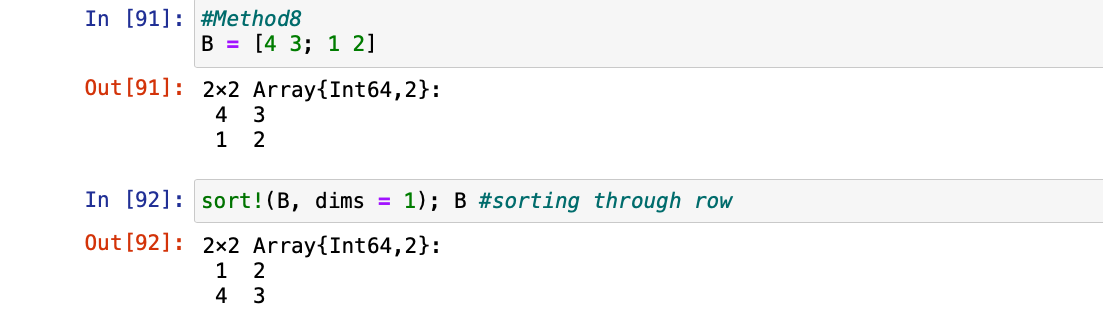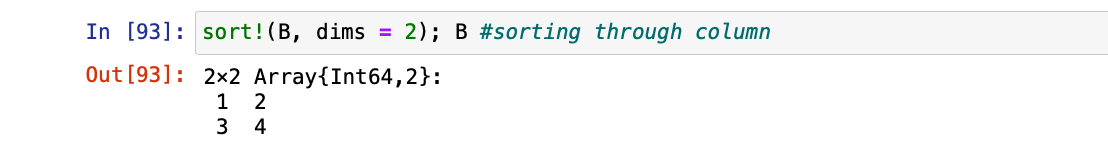My Personal Notes arrow_drop_up